Script 3.3. of the online course "An introduction to agent-based modeling with Python" by Claudius Gräbner

For the course homepage and links to the acompanying videos (in German) see: http://claudius-graebner.com/introabmdt.html

For the Enlish version (currently without videos) see: http://claudius-graebner.com/introabmen.html

Last updated July 18 2019

# Introduction¶

Plots in Python are usually created using the matplotlib library. It is a collection of many modules that allow you to complete basically every visualization task you might think of.

In :
import numpy as np
import pandas as pd
import matplotlib.pyplot as plt
# The following is not necessary for you, only for me creating the html
% matplotlib inline


# The two different approaches to plotting¶

Matplotlib offers you basically two different ways of using it: the procedural API, which resembles a popular programming languages called MATLAB, and an object-oriented API, which follows more closely the typical Python logic.

By the way, API stands for 'Application Programming Interface', and it is basically the interface through which you interact with the program.

## The pyplot API¶

This is a procedural API that resembles MATLAB syntax.

Lets consider an example, where you have data on a time series, stored as an array. The values in the time series is what we would like to plot on the vertical ('y') axis. For the horizontal axis we create an appropriate index vector:

In :
y_axis_data = np.random.random(50)
x_axis_data = np.linspace(1, 50, 50)
plt.plot(x_axis_data, y_axis_data)

Out:
[<matplotlib.lines.Line2D at 0x10fafc3c8>]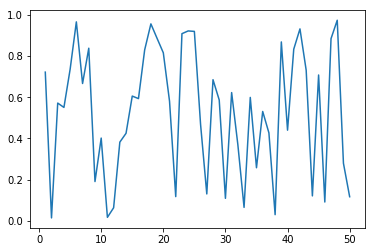This kind of command is very simple, and useful for quick-and-dirty data explorations.

## The object-oriented API¶

The object-oriented API resembles more closely the logic of Python and provides you much more freedom in specifying the plots.

To create the same figure as above we proceed as follows:

In :
fig, ax = plt.subplots()
ax.plot(x_axis_data, y_axis_data)

Out:
[<matplotlib.lines.Line2D at 0x10a0ca940>]Here we first two instances of the classes figure and axes:

In :
print(type(fig))
print(type(ax))

<class 'matplotlib.figure.Figure'>
<class 'matplotlib.axes._subplots.AxesSubplot'>


Then we do operations on them. fig is a blank canvas, it is always in the background. ax is the axes, the actual plot. It is not the same as an axis!. Since everything gets drawn here, this is where we call the method plot from.

This way, it is very easy to process the figure further, and make it better looking.

(You can finde a nice overview over all parts of plots here).

## Conclusion¶

While the object-oriented API provides you with much more functionality and should in general be preferred, the procedural API is useful for some very quick-and-dirty data visualization, e.g. when you try to take your grips on a new data set.

However, to visualize the results of ABM, we generally use the object-oriented variant, which is why we will stick to this kind of syntax in what follows.

# Examples¶

Plotting is best learned by example. Therefore, we discuss three examples in a bit more detail. At the end, I provide you with a link to the Matplotlib Gallery, where you find the code for many example application. After this short intro, you should be able to understand most of it and to be able to build your own visualizations.

## Visualize a single time series¶

Suppose we have data on a single time series, either from a result of an ABM, or because we have, say, downloaded data on the dynamics of the Gini coefficient in Germany.

In both cases, we have the data as either a list or a pd.DataFrame:

In :
ts_data_list = np.random.random(101) # ts data given as list
ts_data_frame = pd.DataFrame({"total_wealth":ts_data_list,
"total_consumption": np.random.random(len(ts_data_list)),
"time":np.linspace(0, 100, len(ts_data_list), dtype=int)})

Out:
time total_consumption total_wealth
0 0 0.727886 0.755509
1 1 0.777741 0.597181

We now build the final plot step by step. The first thing to do is to create the axes and figure instances. This can be done with the plt.subplots function. As arguments we provide the number of subplots. Since we only want to have a single plot we stick with the defauls value '1'.

As with regard to the figure size: having the plot about $1.33$ times wider than tall for each row of plots is a good rule of thumb. Since we only have one plot we choose the size (8,6).

In :
fig, ax = plt.subplots(figsize=(8,6))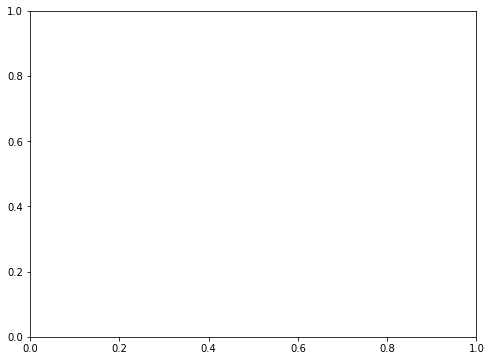Next we plot the time series by supplying both the values for the x and y axis:

In :
fig, ax = plt.subplots(figsize=(8,6))
ax.plot(x_axis_data, y_axis_data)

Out:
[<matplotlib.lines.Line2D at 0x112f78a58>]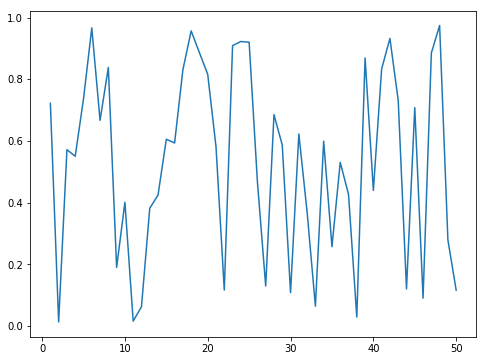It is almost always a good idea to remove unnecessary parts of the plot. For example, the frame lines and the axis ticks on the right and top of the plot are just distracting and not necessary.

In :
fig, ax = plt.subplots(figsize=(8,6))
ax.plot(x_axis_data, y_axis_data)
ax.spines["top"].set_visible(False) # Remove plot frame line on the top
ax.spines["right"].set_visible(False) # Remove plot frame line on the right

ax.get_xaxis().tick_bottom() # ticks of x axis should only be visible on the bottom
ax.get_yaxis().tick_left()  # ticks of < axis should only be visible on the left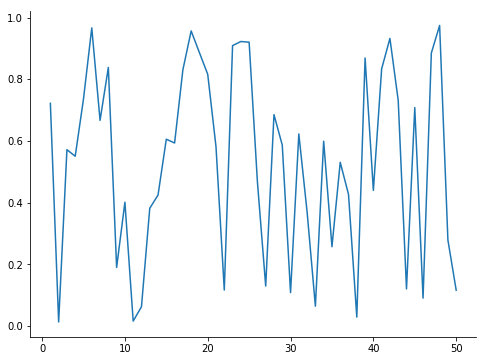We may add grid lines, at least for the y axis. The lines should, however, be rather transparent and not too distracting. Whenever you use a grid, you should remove the axis ticks, since the grid makes them superflouus.

In :
fig, ax = plt.subplots(figsize=(8,6))
ax.plot(x_axis_data, y_axis_data)
ax.spines["top"].set_visible(False) # Remove plot frame line on the top
ax.spines["right"].set_visible(False) # Remove plot frame line on the right

ax.get_xaxis().tick_bottom() # ticks of x axis should only be visible on the bottom
ax.get_yaxis().tick_left()  # ticks of < axis should only be visible on the left

ax.yaxis.grid(color='grey',  # plot grid in grey
linestyle='-', # use normal lines
linewidth=1,   # the width should not be too much
alpha=0.45)    # transparent grids look better

plt.tick_params(axis="both", which="both", bottom="off", top="off",
labelbottom="on", left="off", right="off", labelleft="on")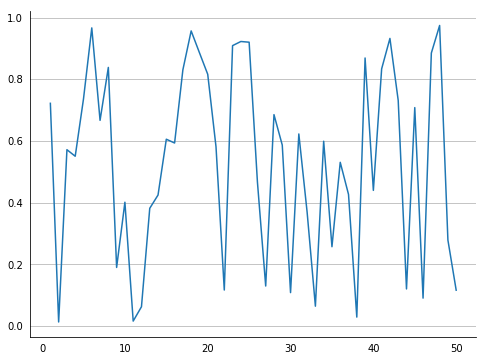Now we should also add a title and labels for the x and y axis:

In :
fig, ax = plt.subplots(figsize=(8,6))
ax.plot(x_axis_data, y_axis_data)
ax.spines["top"].set_visible(False) # Remove plot frame line on the top
ax.spines["right"].set_visible(False) # Remove plot frame line on the right

ax.get_xaxis().tick_bottom() # ticks of x axis should only be visible on the bottom
ax.get_yaxis().tick_left()  # ticks of < axis should only be visible on the left

ax.yaxis.grid(color='grey',  # plot grid in grey
linestyle='-', # use normal lines
linewidth=1,   # the width should not be too much
alpha=0.45)    # transparent grids look better

ax.set_title("Nice plot title")  # The title
ax.set_xlabel("The x axis: time")  # The label for the x axis
ax.set_ylabel("The y axis: random number")  # The label for the y axis

plt.tick_params(axis="both",
which="both",
bottom="off",
top="off",
labelbottom="on",
left="off",
right="off",
labelleft="on")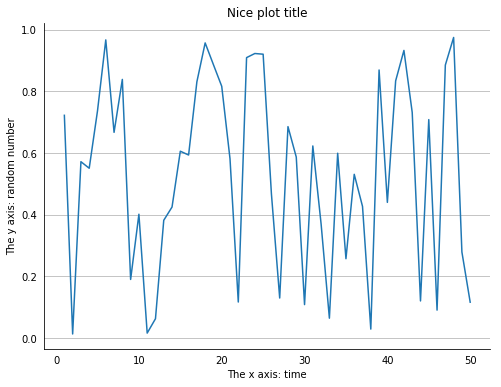Sometimes you might want to add some text on the graph. You can place text using the coordinates of the graph. So, in case you want to place a copyright statement at the bottom of the graph, in our case the y coordinate must be negative.

In :
fig, ax = plt.subplots(figsize=(8,6))
ax.plot(x_axis_data, y_axis_data)
ax.spines["top"].set_visible(False) # Remove plot frame line on the top
ax.spines["right"].set_visible(False) # Remove plot frame line on the right

ax.get_xaxis().tick_bottom() # ticks of x axis should only be visible on the bottom
ax.get_yaxis().tick_left()  # ticks of < axis should only be visible on the left

ax.yaxis.grid(color='grey',  # plot grid in grey
linestyle='-', # use normal lines
linewidth=1,   # the width should not be too much
alpha=0.45)    # transparent grids look better

ax.set_title("Nice plot title")  # The title
ax.set_xlabel("The x axis: time")  # The label for the x axis
ax.set_ylabel("The y axis: random number")  # The label for the y axis

plt.tick_params(axis="both",
which="both",
bottom="off",
top="off",
labelbottom="on",
left="off",
right="off",
labelleft="on")

plt.text(-2.5, -0.25, "Data source: http://phdcomics.com/comics.php?f=1690"
"\nMore fancy text for fancy folks...", fontsize=10)

Out:
Text(-2.5,-0.25,'Data source: http://phdcomics.com/comics.php?f=1690\nMore fancy text for fancy folks...')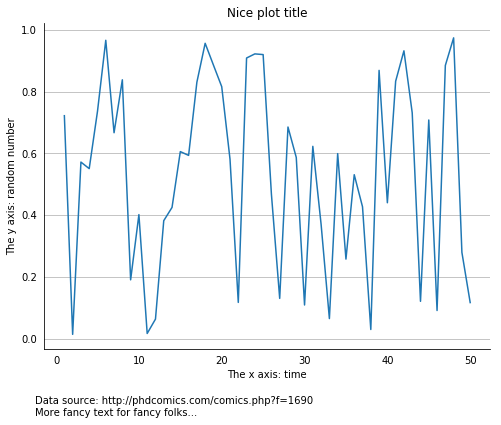Now we just need to save the plot using the plt.savefig command. The format is set via the filename ending. Usually, PDF is the preferred output format.

Finally, you should always add the bbox_inches="tight" argument to remove all the unnecessary whitespace around the plot.

In :
fig, ax = plt.subplots(figsize=(8,6))
ax.plot(x_axis_data, y_axis_data)
ax.spines["top"].set_visible(False) # Remove plot frame line on the top
ax.spines["right"].set_visible(False) # Remove plot frame line on the right

ax.get_xaxis().tick_bottom() # ticks of x axis should only be visible on the bottom
ax.get_yaxis().tick_left()  # ticks of < axis should only be visible on the left

ax.yaxis.grid(color='grey',  # plot grid in grey
linestyle='-', # use normal lines
linewidth=1,   # the width should not be too much
alpha=0.45)    # transparent grids look better

ax.set_title("Nice plot title")  # The title
ax.set_xlabel("The x axis: time")  # The label for the x axis
ax.set_ylabel("The y axis: random number")  # The label for the y axis

plt.tick_params(axis="both",
which="both",
bottom="off",
top="off",
labelbottom="on",
left="off",
right="off",
labelleft="on")

plt.text(-2.5, -0.25, "Data source: http://phdcomics.com/comics.php?f=1690"
"\nMore fancy text for fancy folks...", fontsize=10)

plt.savefig("output/simple_time_series.pdf", bbox_inches="tight")### A version with two subplots¶

Here we build the plot with two subplots, and get the data for the lower plot from the pd.DataFrame.

Here, it is also useful to add plt.tight_layout(True) to the end since this improves space allocation.

In :
fig, axes = plt.subplots(2,1, figsize=(8,12)) # 2,1 means: two rows, one column of plots
axes.plot(x_axis_data, y_axis_data) # we now need to address one of two axes!

axes.spines["top"].set_visible(False) # Remove plot frame line on the top
axes.spines["right"].set_visible(False) # Remove plot frame line on the right

axes.get_xaxis().tick_bottom() # ticks of x axis should only be visible on the bottom
axes.get_yaxis().tick_left()  # ticks of < axis should only be visible on the left

axes.yaxis.grid(color='grey',  # plot grid in grey
linestyle='-', # use normal lines
linewidth=1,   # the width should not be too much
alpha=0.45)    # transparent grids look better

axes.set_title("First plot title")  # The title
axes.set_xlabel("The x axis: time")  # The label for the x axis
axes.set_ylabel("The y axis: random number")  # The label for the y axis

# The lower plot
axes.plot(ts_data_frame["time"],
ts_data_frame["total_wealth"],
linestyle="--")

axes.plot(ts_data_frame["time"],
ts_data_frame["total_consumption"],
linestyle=":")

axes.spines["top"].set_visible(False) # Remove plot frame line on the top
axes.spines["right"].set_visible(False) # Remove plot frame line on the right

axes.get_xaxis().tick_bottom() # ticks of x axis should only be visible on the bottom
axes.get_yaxis().tick_left()  # ticks of < axis should only be visible on the left

axes.yaxis.grid(color='grey',  # plot grid in grey
linestyle='-', # use normal lines
linewidth=1,   # the width should not be too much
alpha=0.45)    # transparent grids look better

axes.set_title("Second plot title")  # The title
axes.set_xlabel("The x axis: time")  # The label for the x axis
axes.set_ylabel("The y axis: random number")  # The label for the y axis

plt.tick_params(axis="both",
which="both",
bottom="off",
top="off",
labelbottom="on",
left="off",
right="off",
labelleft="on")

axes.text(0.0, -0.25, "Data source: http://phdcomics.com/comics.php?f=1690"
"\nMore fancy text for fancy folks...", fontsize=10)

plt.tight_layout(True) # Good to get better alignment

plt.savefig("output/double_time_series.pdf", bbox_inches="tight")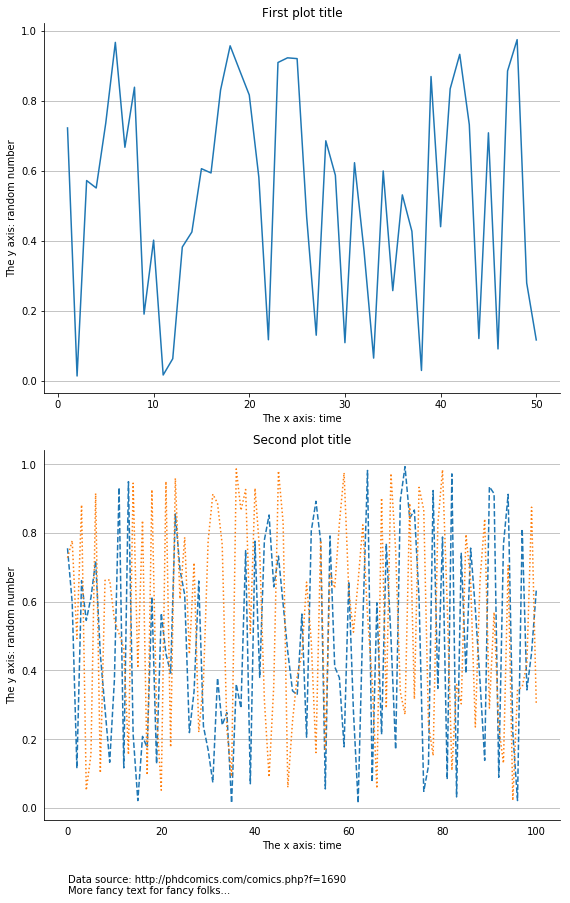## Visualize the mean of many time series¶

When we simulate an ABM we do not simulate it once. Since randomness usually plays a role, every outcome of the ABM is different. Thus, we are more interested in the average behavior or the model, and the degree of variation among different simulations.

Therefore, we create many simulations, take the average and specify the distribution of the outcomes. Thus, we usually do not plot a sinlge time series such as in the example before, but the mean and standard deviation of many time series.

A typical form is that of a pd.DataFrame where one column indicates the particular run of the simulation (here the column id):

In :
expl_frame_loaded = pd.read_feather('data/example_output.feather')

Out:
index id t n_c n_d
0 0 0 0 187 63
1 1 0 1 160 90
2 2 0 2 136 114
3 3 0 3 121 129
4 4 0 4 111 139

Here id stands for the simulation run, t for the time step, and n_c and n_d are state variables of the ABM (which is actually this one).

Thus, we want the averages and standard deviations for n_c and n_d for every t over all different simulations.

To do this, we first group the data by t:

In :
expl_frame_loaded_grouped = expl_frame_loaded.groupby('t')


Then we aggregate the data, and return means and standard variations for the variables n_c and n_d.

In :
expl_frame_loaded_plot = expl_frame_loaded_grouped.agg([np.mean, np.std])[["n_c", "n_d"]]

Out:
n_c n_d
mean std mean std
t
0 187.00 0.000000 63.00 0.000000
1 163.58 6.158087 86.42 6.158087
2 141.58 7.969559 108.42 7.969559
3 124.50 9.978037 125.50 9.978037
4 112.40 11.559959 137.60 11.559959

We are now ready to plot the data. As above we start by creating instances for the figure and the axes, and we remove superflous axis lines and ticks:

In :
fig, ax = plt.subplots(figsize=(12,9)) # 2,1 means: two rows, one column of plots

ax.spines["top"].set_visible(False) # Remove plot frame line on the top
ax.spines["right"].set_visible(False) # Remove plot frame line on the right

ax.get_xaxis().tick_bottom() # ticks of x axis should only be visible on the bottom
ax.get_yaxis().tick_left()  # ticks of < axis should only be visible on the left

ax.yaxis.grid(color='grey',  # plot grid in grey
linestyle='-', # use normal lines
linewidth=1,   # the width should not be too much
alpha=0.45)    # transparent grids look better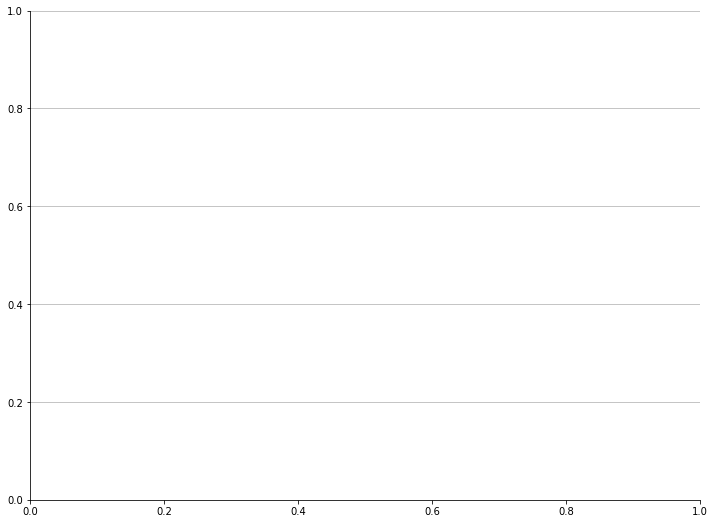We now add the time series for the mean to the plot:

In :
fig, ax = plt.subplots(figsize=(12,9)) # 2,1 means: two rows, one column of plots

ax.spines["top"].set_visible(False) # Remove plot frame line on the top
ax.spines["right"].set_visible(False) # Remove plot frame line on the right

ax.get_xaxis().tick_bottom() # ticks of x axis should only be visible on the bottom
ax.get_yaxis().tick_left()  # ticks of < axis should only be visible on the left

ax.yaxis.grid(color='grey',  # plot grid in grey
linestyle='-', # use normal lines
linewidth=1,   # the width should not be too much
alpha=0.45)    # transparent grids look better


Out:
[<matplotlib.lines.Line2D at 0x11353d2e8>]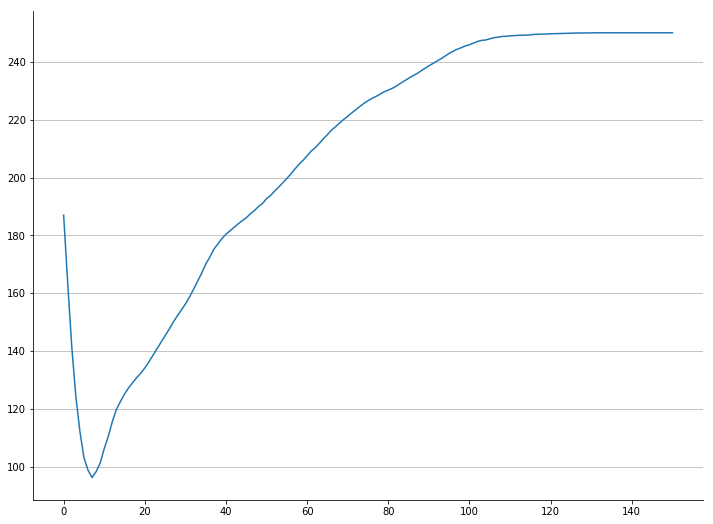We now want to visualize the variation across simulation runs using a shaded area around the mean. We use the method fill_between: its first argument is the data for the x axis, then we have to define two trajectories that indicate the upper and lower bound for the shaded area. This will be the mean plus/minus one standard deviation.

In :
fig, ax = plt.subplots(figsize=(12,9)) # 2,1 means: two rows, one column of plots

ax.spines["top"].set_visible(False) # Remove plot frame line on the top
ax.spines["right"].set_visible(False) # Remove plot frame line on the right

ax.get_xaxis().tick_bottom() # ticks of x axis should only be visible on the bottom
ax.get_yaxis().tick_left()  # ticks of < axis should only be visible on the left

ax.yaxis.grid(color='grey',  # plot grid in grey
linestyle='-', # use normal lines
linewidth=1,   # the width should not be too much
alpha=0.45)    # transparent grids look better

ax.fill_between(np.arange(0, 151, 1),
color="#3F5D7D")
ax.plot(np.arange(0, 151, 1), expl_frame_loaded_plot["n_c"]["mean"], color="white", lw=2)

Out:
[<matplotlib.lines.Line2D at 0x1132b6c50>]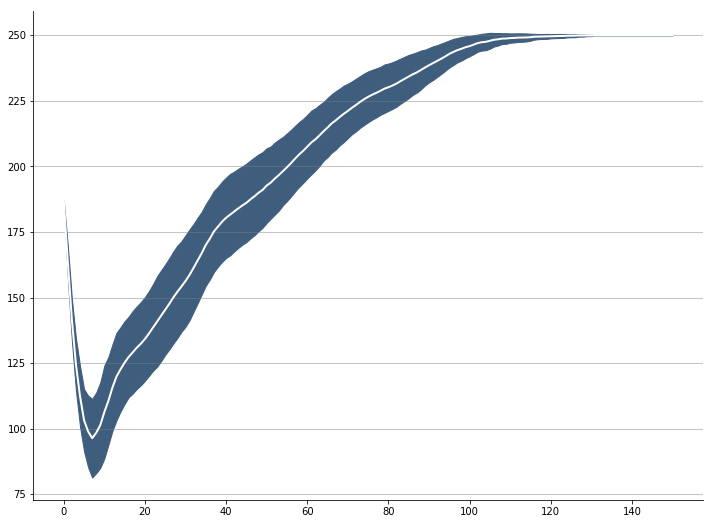This looks nice! Now we create the same figure, but with two subplots, one for each of the variables. Then we add titles, call plt.tight_layout(True) and save the plot - again using bbox_inches="tight".

In :
fig, axes = plt.subplots(2, 1, figsize=(12,9)) # 2,1 means: two rows, one column of plots

# The first subplot

axes.spines["top"].set_visible(False) # Remove plot frame line on the top
axes.spines["right"].set_visible(False) # Remove plot frame line on the right

axes.get_xaxis().tick_bottom() # ticks of x axis should only be visible on the bottom
axes.get_yaxis().tick_left()  # ticks of < axis should only be visible on the left

axes.yaxis.grid(color='grey',  # plot grid in grey
linestyle='-', # use normal lines
linewidth=1,   # the width should not be too much
alpha=0.45)    # transparent grids look better

axes.fill_between(np.arange(0, 151, 1),
axes